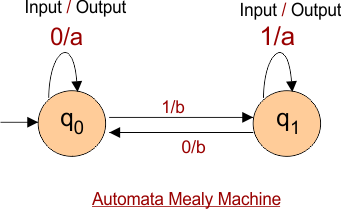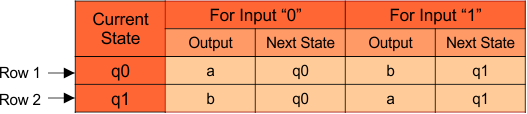Select Page

# Automata Mealy Machine

Automata Mealy Machine is just similar to Automata Moore Machine except the following,

• In the Automata Mealy Machine for each state, the output symbol is represented along with input symbol. Both input and output symbols are separated by /.
• And in Mealy Machine length of input is always equal to output. Because In Mealy Machine first output comes by consuming first input symbol.
• Before to reach the next state, Output receive immediately as the input consumed.

An example of Mealy Machine is given below## Tuples of Mealy Machine

Mealy Machine can be described by 6 tuples (Q, q0, ∑, O, δ, λ’). Let explain above Mealy Machine where

• q0 and q1 are states (Q).
• q0 is the initial state.
• Input alphabets (∑) are “0” and “1”.
• Output alphabets (O) are “a” and “b”.
• Transition function (Q × ∑ →Q) and output function (Q × ∑ →O) are explained in the following table

## Transition Table in Mealy Machine

In Automata Mealy Machine, When the input is given then Mealy Machine first gives an output and then goes to next state.

In the above given Mealy Machine, the transition table is given belowIn Row 1

• When the given Input is “0” at initial state (q0) then output will be “a” and Next state is also “a”.
• Similarly, When the given Input is “1” at initial state (q0) then output will be “b” and Next state will be “q1”

In Row 2

• When the given Input is “0” at initial state (q1) then output will be “b” and Next state is also “b”.
• Similarly, When the given Input is “1” at initial state (q1) then output will be “a” and Next state will also “q1”

Note: Length of output will always equal to length of input.

In next lecture we will see the example of Automata Mealy Machines.

Help Other’s By Sharing…